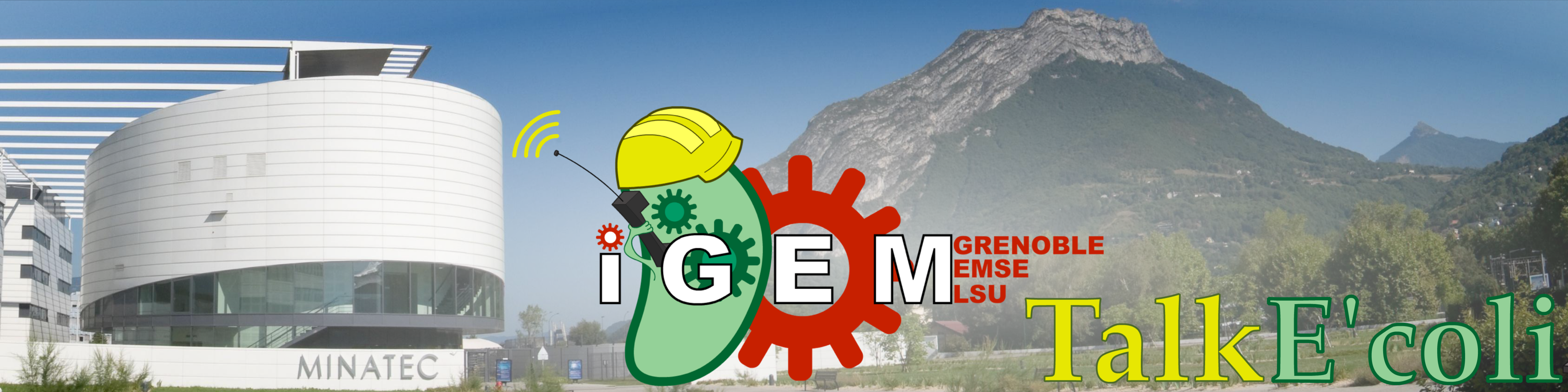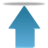# Team:Grenoble-EMSE-LSU/Project/Modelling/Parameters

Grenoble-EMSE-LSU, iGEMGrenoble-EMSE-LSU, iGEM

• # Finding parameters

Our model now considers the maturation of KillerRed and the accumulation of damages done to the bacteria. It is able to describe the evolution of all three quantities that are observed: the optical density of the suspension, its fluorescence and the density of living cells. But we still have to find suitable parameter values to reproduce the experimental data and to simulate the model.

$r$: the rate of growth of bacteria in $min^{-1}$

$a$: the production of KillerRed per bacteria in $RFU.OD^{-1}.min^{-1}$

$b$: the efficiency of photobleaching in $RFU.UL^{-1}.min^{-1}$

$m$: the maturation rate of KillerRed in $min^{-1}$

$k$: the toxicity of KillerRed in $OD.RFU^{-1}.UL^{-1}.min^{-1}$

$l$: the rate of healing of the bacteria by step of time. unit less

With the units :

$OD$ is the Optical Density at $\lambda = 600nm$

$RFU$ is Relative Fluorescent Units (with $\lambda_absorption=585nm$ and $\lambda_emission=610nm$

$UL$ is an arbitrary Unit of Light, related to the energy received by the bacteria. $1 UL$ shall be the energy of light received by an Erlenmeyer flask with a MR16 LED on its side at full power.

The aim is to find the set of parameters that best fits the curves of $OD_{600}$ and fluorescence observed. As we cannot determine them separately because they have opposite effects, we searched for the set of parameters that minimizes the distance between the outputs of the model and the experimental data. The distance chosen is the Euclidian distance : the Sum of Square Residuals, or SSR. In our case, the easiest and quickest methods are unusable:

$\diamond$ A regression requires the solutions to be analytic functions, such as polynomials or exponentials to project the points on it.

$\diamond$ Gradient or Newton methods require regularity in the effect of parameters that we do not have.

$\diamond$ The technique of experimental design is not usable for the same reason.

We therefore used an alternative method based on the utilization of Genetic Algorithms.

• ## Genetic Algorithms

At first sight, the only way to find our parameters was to adjust them manually until the model predictions fitted the experimental data. It wasn't a slow method since we could imagine how the output of the calculations would change when we varied each parameter. However this did not ensure that the best solution was found. This information can be obtained by exhaustive research, but this is a pretty long process. To verify 10 values for each parameter, $10^6$ tests are needed, and each test consists in the calculation of 1000 points. For a standard computer, this represents 2 hours of continuous processing. Considering that 10 values are not enough to get a precise answer, this approach would have been difficult to use in practice.

This is the reason why we used genetic algorithms.

The idea of a genetic algorithm is based on the evolution of a wild population and the natural selection of phenotypes best adapted to environment. Here, a phenotype is a set of parameters, and the measure of adaptation is the distance of the kinetics predicted from the kinetics observed.

1. First we start with a randomly chosen population (not too random to accelerate the process).

2. The best ones; those that minimize the distance between previsions and observations, are selected.

3. With these best ones, other phenotypes are created by mixing the values of parameters (crossing-over) and modifying some of them a bit (mutations).

4. We now have a second generation population. If they are all close enough to the solution (ie, the distance between previsions and observations is small enough), the algorithm is considered as 'stabilized', the best one is chosen and the process stops. If not, the algorithm goes back to step 2 with these new phenotypes.

For 6 parameters, this genetic algorithm works well with a population of 21 phenotypes and 6 selected ones to breed the next generation. To be sure to explore a lot of sets of parameters, we don't choose the 6 best ones, but we choose 5 out of the 6 best ones randomly, and 1 out of the 15 others. It makes the process longer but creates better solutions.

As this algorithm is not deterministic, the only way to compare it to the exhaustive research is to stay in front of the computer with a chronometer. The time gain is quite good : in only five minutes, we have a much more precise result.

• ## Results

We ran 5 experiments to find our parameters. All with the same start procedure, but with different kinds of illumination :

$\bullet$In the 3 first ones, the light is switched off for the first 200 minutes, then switched on at full power.

$\bullet$In the 2 last ones, the light is switched off for the 180 first minutes, then switched on at full power, and then we modified the intensity of the light several times in order to stabilize the bacterial growth with the help of the predictions. We have succeeded in stabilizing the last one, but not the other.

The 3 first experiments also permitted us to be sure our procedure was repeatable. And the two others, with a variable light, allowed us to be sure our parameters are independent from variations in the environment and to show that the model reacts properly to different intensities of light.

For each experiment, we searched for the best parameters.

table of parameters
exp 1 exp 2 exp 3 exp 4 exp 5 mean Standard Deviation Standard Score $\left(\frac{\sigma}{\mu}, in \%\right)$
$R$ 75 82 81 81 95 82.8 7.36 8.89
$a$ 93 150 150 170 100 132 34.0 25.7
$b (.10^{-2})$ 1.5 1.0 0.8 0.3 0.7 0.86 0.439 51.1
$M$ 120 100 100 120 100 108 11.0 10.1
$k (.10^{-7})$ 1.1 1.5 1.1 0.15 0.5 0.87 0.538 61.8
$1-l$ 0.004 0.02 0.03 0.018 0.011 0.0166 0.00979 59.0

$M$ and $R$ are not the variables used in the equation, but are directly related to them :

$R$ is the time of division of bacteria, and so : $r=\frac{\ln(2)}{R}$.

$M$ is the time at which half of the KillerRed proteins have matured, and so : $m=\frac{\ln(2)}{M}$ .

$M$ and $R$ are both actual times (in $min$) and make the figures easier to understand.

In the table, $1-l$ appears, and not $l$ for $l$ has a huge effect on the previsions when $l\rightarrow1$. Turning it that way allows us to better control its variations, a variation of 1% of $l$ can make it exceed the value $1$, which becomes meaningless, whereas for $1-l$ even a variation of 10% does not make it exceed any limit value.

Analyzing the table shows that $M$ and $R$ are the least variable parameters. In contrast, $b$, $k$ and $l$, the parameters that characterize KillerRed photobleaching, photo toxicity and damage accumulation exhibit large variations. These parameters should therefore be determined in each experiment, by a standard procedure described here.

The mean values will be the ones used for the predictions, except for the 3 volatile parameters : their mean value will be used to begin with, but when, during the experiment, it is possible to improve the precision of these mean values, they are changed.